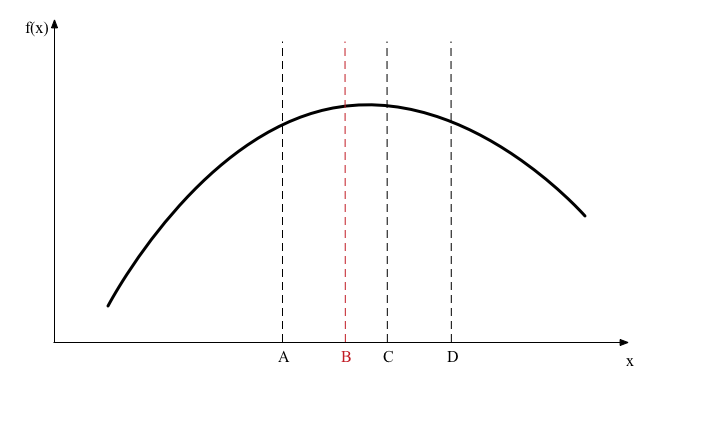# Numerical maximization¶

In the Bellman equation we maximize over $$(C,K')$$. By substituting the aggregate resource constraint we can phrase this as a maximization over one choice variable
$\begin{split}V(K,Z) &= \max_{K'} \left\{ u\left( f(K,Z) - K' \right) + \beta \mathbb E V(K',Z') \right\}.\end{split}$

Optimization is a large field of numerical analysis, but in these notes we will consider just one method that is easy to understand and fairly robust called “golden section search”. Suppose we have a function $$F(x)$$ and we want to maximize it on the interval $$[\underline{x},\bar x]$$. We start by evaluating the function at four points labeled $$A$$, $$B$$, $$C$$, and $$D$$ in the following diagram.To start with we would set $$A = \underline{x}$$ and $$D = \bar x$$. We then observe that $$F(C)>F(B)$$ so we use the following reasoning to discard the interval $$[A,B]$$ from containing the maximizer: if $$F(x)$$ is concave then the derivative is weakly decreasing and if the true maximizer $$x^*$$ were in $$[A,B]$$ then the function goes down from $$x^*$$ to B and then up from B to C, which contradicts the supposition that $$f$$ is concave. You could use this algorithm if $$f$$ is not concave but then there are no guarantees that it will work.

Once we eliminate the interval $$[A,B]$$ we repeat the same steps on the interval $$[B,D]$$ that is smaller than our original interval $$[A,D]$$. So point $$B$$ will become the new $$A$$. The clever part of the algorithm is that we put the points $$B$$ and $$C$$ in just the right places so that now $$C$$ becomes the new $$B$$. This means we don’t need to re-evaluate the function at our new points $$A$$ or $$B$$. We now introduce a new point $$C$$, evaluate the function there and repeat as shown in the next diagram.In the next iteration we find $$F(B)>F(C)$$ so now we discard $$[C,D]$$, $$C$$ becomes the new $$D$$, and $$B$$ becomes the new $$C$$.As you can see in the diagrams, the region under consideration is shrinking towards the true maximizer. If we repeat this procedure many times over we will have $$[A,D]$$ tightly bracketing $$x^*$$.

Finally, how do we choose the points $$B$$ and $$C$$? We are going to impose some symmetry on the choice so the interval $$[A,B]$$ is the same length as $$[C,D]$$. This reduces the problem to choosing one number $$p \in (0,1)$$ as shown in the following figure.We want to choose $$p$$ so that when we eliminate $$[A,B]$$ point $$C$$ becomes the new point $$B$$ so we need the ratio of $$BC$$ relative to $$BD$$ to be the same as $$AB$$ relative to $$AD$$. To accomplish this we need
$\frac{2p-1}{p} = 1-p.$

This equation reduces to a quadratic equation with one root $$p = (-1 + \sqrt{5})/2$$. As the other root implies $$p<0$$ we disregard it.

Why is the method called “golden section search”? Because $$1/p$$ is the golden ratio.